International
Tables for
Crystallography
Volume C
Mathematical, physical and chemical tables
Edited by E. Prince

International Tables for Crystallography (2006). Vol. C, ch. 1.2, pp. 7-9

Section 1.2.5. Trigonal and hexagonal crystal system

E. Kocha

aInstitut für Mineralogie, Petrologie und Kristallographie, Universität Marburg, Hans-Meerwein-Strasse, D-35032 Marburg, Germany

1.2.5. Trigonal and hexagonal crystal system

| top | pdf |

1.2.5.1. Description referred to hexagonal axes

| top | pdf |

Metrical conditions: a = b; c arbitrary; α = β = 90°; γ = 120°

Bravais lattice types: hP, hR

Symmetry of lattice points: 6/mmm (hP),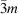(hR)

 Table 1.2.5.1| top | pdf | Assignment of integersto pairs h, k with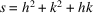Each pair h, k represents in addition the pairs k, −h − k and −h − k, h, the permutations of these three, and the six corresponding centrosymmetrical pairs.
shkshkshk
1 1 0 31 5 1 67 7 2
3 1 1 36 6 0 73 8 1
4 2 0 37 4 3 75 5 5
7 2 1 39 5 2 76 6 4
9 3 0 43 6 1 79 7 3
12 2 2 48 4 4 81 9 0
13 3 1 49 7 0 84 8 2
16 4 0 5 3 91 9 1
19 3 2 52 6 2 6 5
21 4 1 57 7 1 93 7 4
25 5 0 61 5 4 97 8 3
27 3 3 63 6 3 100 10 0
28 4 2 64 8 0

1.2.5.2. Description referred to rhombohedral axes

| top | pdf |

Metrical conditions: a = b = c; α = β = γ

Bravais lattice type: hR

Symmetry of lattice points:Simplified formulae: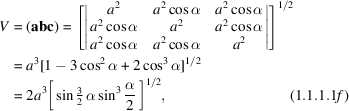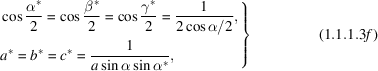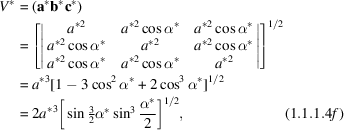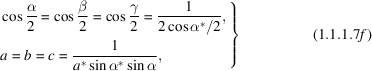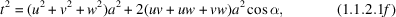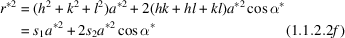with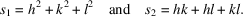For each value of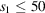, all corresponding values of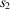and all triplets h, k, l are listed in Table 1.2.5.2.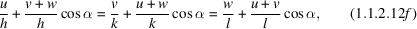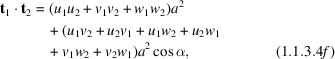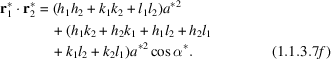Table 1.2.5.2| top | pdf | Assignment of integersto triplets h, k, l withand to integers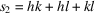Each triplet h, k, l represents all twelve triplets resulting from permutation and/or simultaneous change of all signs.
s1s2hkls1s2hkls1s2hkl
1 0 1 0 0 24 −12 −4 2 2 38 −19 −5 3 2
2 −1 −1 1 0 −4 4 −2 2 −11 −6 1 1
1 1 1 0 20 4 2 2   5 −3 2
3 −1 −1 1 1 25 −12 −4 3 0 −1 6 −1 1
3 1 1 1 0 5 0 0   5 3 −2
4 0 2 0 0 12 4 3 0 13 6 1 1
5 −2 −2 1 0 26 −13 −4 3 1 31 5 3 2
2 2 1 0 −11 4 −3 1 40 −12 −6 2 0
6 −3 −2 1 1 −5 −5 1 0 12 6 2 0
−1 2 −1 1 5 5 1 0 41 −20 −5 4 0
5 2 1 1   4 3 −1 −16 −6 2 1
8 −4 −2 2 0 19 4 3 1   −4 4 3
4 2 2 0 27 −9 −5 1 1 −8 6 −2 1
9 −4 −2 2 1   −3 3 3   4 4 −3
0 3 0 0 −1 5 −1 1 4 6 2 −1
2 2 −1 11 5 1 1 20 6 2 1
8 2 2 1 27 3 3 3   5 4 0
10 −3 −3 1 0 29 −14 −4 3 2 40 4 4 3
3 3 1 0 −10 −5 2 0 42 −21 −5 4 1
11 −5 −3 1 1   4 −3 2 −19 5 −4 1
−1 3 −1 1 −2 4 3 −2 11 5 4 −1
7 3 1 1 10 5 2 0 29 5 4 1
12 −4 −2 2 2 26 4 3 2 43 −21 −5 3 3
12 2 2 2 30 −13 −5 2 1 −9 5 −3 3
13 −6 −3 2 0 −7 5 −2 1 39 5 3 3
6 3 2 0 3 5 2 −1 44 −20 −6 2 2
14 −7 −3 2 1 17 5 2 1 −4 6 −2 2
−5 3 −2 1 32 −16 −4 4 0 28 6 2 2
1 3 2 −1 16 4 4 0 45 −22 −5 4 2
11 3 2 1 33 −16 −5 2 2 −18 −6 3 0
16 0 4 0 0   −4 4 1   5 −4 2
17 −8 −3 2 2 −4 5 −2 2 2 5 4 −2
−4 −4 1 0 8 4 4 −1 18 6 3 0
3 −2 2 24 5 2 2 38 5 4 2
4 4 1 0   4 4 1 46 −21 −6 3 1
16 3 2 2 34 −15 −5 3 0 −15 6 −3 1
18 −9 −3 3 0   −4 3 3 9 6 3 −1
−7 −4 1 1 −9 4 −3 3 27 6 3 1
−1 4 −1 1 15 5 3 0 48 −16 −4 4 4
9 4 1 1 33 4 3 3 48 4 4 4
3 3 0 35 −17 −5 3 1 49 −24 −6 3 2
19 −9 −3 3 1 −13 5 −3 1 −12 6 −3 2
3 3 3 −1 7 5 3 −1 0 7 0 0
15 3 3 1 23 5 3 1   6 3 −2
20 −8 −4 2 0 36 −16 −4 4 2 36 6 3 2
8 4 2 0 0 6 0 0 50 −25 −5 5 0
21 −10 −4 2 1   4 4 −2 −23 −5 4 3
−6 4 −2 1 32 4 4 2 −17 5 −4 3
2 4 2 −1 37 −6 −6 1 0 −7 −7 1 0
14 4 2 1 6 6 1 0   5 4 −3
22 −9 −3 3 2         7 7 1 0
−3 3 3 −2         25 5 5 0
21 3 3 2         47 5 4 3